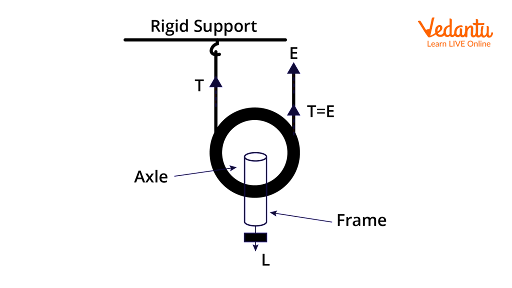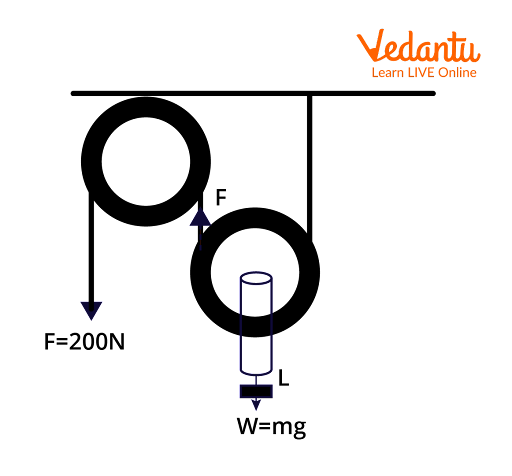Courses
Courses for Kids
Free study material
Offline Centres
More

# Single Movable Pulley - Important Concepts With Examples for JEELast updated date: 01st Dec 2023
Total views: 22.5k
Views today: 1.22k## Insights Into Pulleys

In our lives, there is a prominent usage of pulleys in many industrial types of machinery and marine equipment where there is an essential need of transferring heavy objects and pulling heavy weights. These have the mechanical advantage of applying heavy forces on the objects. A typical pulley looks like a wheel which is compacted on a shaft for the movement of the rope tied to it.

A pulley's operation method is straightforward and can be easily apprehended. Before the usage of any pulley, it is presumed that the weight of the pulley and the weight of the rope is not taken into consideration. Only the weight of the load block which is attached to the pulley is considered and calculated. Here, the pulley and the rope are in contact with each other, so there must be friction present between those two objects. But we assume that there is no loss of energy due to that friction and we also assume that the rope is not stretchable. Let us dive into the concept of what a pulley is, the pulley system including the movable pulley’s mechanical advantage and pulley examples.

## Types of Pulleys

In essence, pulleys are divided into three types on the basis of the movement of the pulleys and the structure of the pulley system with a certain number of pulleys operating in it. They are:

• Fixed

• Movable and

• Compound pulleys

A fixed pulley is a type of pulley in which the shaft or axle is fixed on support and only the rope is moving according to the force applied to it. In a fixed pulley, the mechanical advantage is the least and to increase it we need to combine two fixed pulleys together. Importantly, the mechanical advantage of a system could be described as the ratio of the force produced by the machine in order to work and the force applied to the machine. This mechanical advantage is widely termed and used to assess the performance and efficiency of the system.

A movable pulley system consists of a pulley whose axis of rotation is not constant. When there is a combination of fixed and movable pulleys mounted together, then the system of pulleys is defined as a compound pulley. Let us learn the main concept of a single movable pulley and pulley examples in detail.

## Single Movable Pulley

A movable pulley is a type of pulley in which the shaft or axle is attached to the moving block and one end of the rope is attached to a support. Whereas the other end can be left free and now there exists only a single pulley in the system and moreover, it is movable. This system is defined as a single movable pulley and it looks like as shown in the figure.Single Movable Pulley

Here, The Load force acting on the block in the downward direction is L and the tension in the string on the left is T. Here, the other end of the string would be used to apply a pulling effort through which the block is lifted up or lifted down. This effort will come from the tension applied on the string and the ratio of load force to the pulling effort is known as mechanical advantage.

Mechanical advantage $=\dfrac{\text{load}}{\text{Effort}}$

Therefore, the mechanical advantage of the above shown mechanical pulley is given by the equation,

$MA=\dfrac{L}{E}$

Where at the equilibrium position the forces acting on the block and the tension in the string remain with a constant ratio.

Therefore, L = T + T = 2T = 2E

$\mathrm{MA}=\frac{L}{E}=\frac{2 E}{E}=2$

As a single movable pulley is operated with two ends of the same rope, so it has a mechanical advantage for the two. Let us understand the movable pulley concept through example problems.

## Solved Examples on Single Movable Pulleys

Examples 1: Find the mechanical advantage of a movable compound pulley shown in the below figure whose mass of the load is 40kg and the force applied to lift the load is 200N.Compound Pulley Example

Solution:

Given that, the Mass of the load attached to the pulley is, m = 25kg.

Weight of the load acting towards the ground, W = mg = 40 $\times$ 10 = 400N

Therefore, the load force is 400N and the effort of force applied is, E = 200N.

Therefore, the mechanical advantage is given by, $MA=\dfrac{L}{E}=\dfrac{400}{200}=2$.

Example 2: In a pulley system, given that the mechanical advantage = 4 and the Effort applied is 25N. Find the mass of the load in the system.

Solution:

This is an example of a movable pulley.

We know that, $MA=\dfrac{L}{E}$

Given that MA = 4 and E = 25N

Therefore, we know that MA $\times$ E = L

Therefore, L = 4 $\times$ 25 = 100N

L = mg = 100N

I.e., m $\times$ 10 = 100

Therefore, the mass of the load is 10kg.

## Conclusion

We discussed what a pulley is and how a single movable pulley works. We described the mechanical advantage of the single movable pulley with its formula and pulley examples. The main use of pulleys is that they make lifting and transferring things easier. These are widely used in various applications and the use of pulley is vital in marine and shipping operations too.

## FAQs on Single Movable Pulley - Important Concepts With Examples for JEE

1. What are the applications of a pulley in our daily lives?

The use of pulleys makes a wider impact on various applications which have an operation of lifting weights. This pulley system is used and regularly seen in borewells where the water is filled in a small bucket by passing it through a rope fixed on a pulley. In many exercise equipment, there is an application of pulleys in machinery also. These are used in elevators, construction procedures, flagpoles, cranes and in escalators. A pulley is a simple and vivid machine that makes many things easier for humans.

2. What are the types of pulley systems?

There are three types of pulley systems which are prominent and widely used in all the applications of pulley systems. These are fixed pulley, movable pulley and compound pulley. Fixed pulleys are those whose axis of rotation remains constant as it is fixed to the support in the system. In a movable pulley, the axis of rotation is not constant and the pulley is attached to the block part of the system. Whereas, the compound pulley is the combination of both the fixed and movable pulleys.